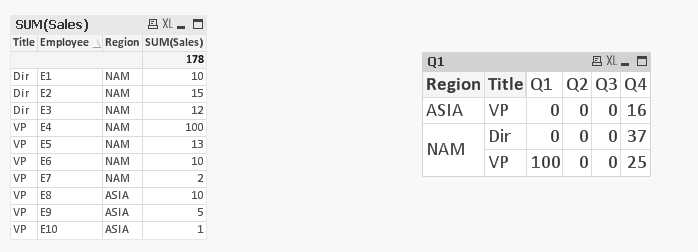# New to QlikView

Discussion board where members can get started with QlikView.

Announcements
Coming your way, the Qlik Data Revolution Virtual Summit. October 27-29. REGISTER
cancel
Showing results for
Did you mean:
HighlightedNot applicable

## Quartile metric calculation using set analysis

Hi All,

I need to create a pivot table by grouping the metric (sales) into 4 groups (Quartiles).

Q1 - Sum of all sales where Sales > (Max(Sales)*0.75)

Q2 - Sum of all sales where Sales >= (Max(Sales)*0.5) and Sales < (Max(Sales)*0.75)

Q3 - Sum of all sales where Sales >= (Max(Sales)*0.25) and Sales < (Max(Sales)*0.5)

Q4 - Sum of all sales where Sales < (Max(Sales)*0.25)

e.g: For NAM and Dir combination Max(Sales) is 15 and Max(Sales)*0.75 is 11.25

Q1= 15+12 =27

Q2= 10

Q3=0

Q4=0

Data set

 Region Title Employee Sales NAM Dir E1 10 NAM Dir E2 15 NAM Dir E3 12 NAM VP E4 16 NAM VP E5 13 NAM VP E6 10 NAM VP E7 2 ASIA VP E8 10 ASIA VP E9 5 ASIA VP E10 1

Expected Output:

 Region Title Q1 Q2 Q3 Q4 NAM Dir 27 10 0 0 NAM VP 29 10 0 2 ASIA VP 10 5 0 1

I tried below expression but does not produce correct results.

Q1= sum ({\$<Sales={'>= \$(#=max(Sales)*0.75)'}>}Sales)

Q2= sum ({\$<Sales={'< \$(#=max(Sales)*0.75) >= \$(#=max(Sales)*0.5) '}>}Sales)

Q3= sum ({\$<Sales={'< \$(#=max(Sales)*0.5) >= \$(#=max(Sales)*0.25) '}>}Sales)

Q4= sum ({\$<Sales={'< \$(#=max(Sales)*0.25)'}>}Sales)

1 Solution

Accepted Solutions
HighlightedCreator II

Try the following expressions:

Q1:

Sum(Aggr(If(Sum(Sales) >= Max(TOTAL <Title,Region> Sales) * 0.75,Sum(Sales)),Region,Title,Employee))

Q2:

Sum(Aggr(If(Sum(Sales) >= Max(TOTAL <Title,Region> Sales) * 0.5 And Sum(Sales) < Max(TOTAL <Title,Region> Sales) * 0.75,Sum(Sales)),Region,Title,Employee))

Q3 and Q4 same as these with the factors changed.  This gives the output your after.

It is also customisable so that if your dimensions changes, you can modify the functions to reflect in the appropriate places.

If you introduce other dimensions particularly if they reduce the lowest level below employee may need to play with the functions a little.

16 Replies
HighlightedMVP

My output is slightly different...

Let me know whether it works f

HighlightedPartner

Hi,

I don't think you can use set analysis for this because the set analysis condition is only calculated once for the whole dataset, not per dimension. This means that Max(Sales) inside a set analysis will get the max (=16) for the whole dataset.

You may need to calculate those max values beforehand in the script.

-Teemu

HighlightedNot applicable

Teemu,

Thanks for replying. Is there any other way to achieve this e.g. aggr(), fractlie()?

HighlightedNot applicable

Hi Manish,

appreciate your help. But the xpressions does not appear to work for the reason Teemu has mentioned. I changed the input numbers slightly and reloaded qvw.  Check the output. They are wrong.HighlightedPartner

Hi,

You may be able to use some sort of If statement with aggr etc. but I'm not sure. And personally I hate If statements because they can be very slow. If the row count is not an issue I would probably calculate those dimension specific max values in the script. It would make the expressions a bit simpler too I think.

Sorry but I can't provide you with an example at this time. I'll see if I have time later today.

-Teemu

HighlightedNot applicable

I agree with Teemu, It is efficient to do it in Script.

Other dirty way is to create Variables, But you will end up with 4 * 5 * 3 variables

Assuming 4 regions, 5 Titles and 3 Quartiles. Use those variables in the expression.

It is interesting to know if some one solves this.HighlightedNot applicable

Sudheer, Teemu,

Script may not be an option as I have 30 other dimensions that can change the combination. For e.g. Month, Year, Dept, Region etc.  User can make selection on any of these dimensions and calculation needs to reflect the selections.

I shared a simplified data set for illustrating the problem.

HighlightedCreator II

Try the following expressions:

Q1:

Sum(Aggr(If(Sum(Sales) >= Max(TOTAL <Title,Region> Sales) * 0.75,Sum(Sales)),Region,Title,Employee))

Q2:

Sum(Aggr(If(Sum(Sales) >= Max(TOTAL <Title,Region> Sales) * 0.5 And Sum(Sales) < Max(TOTAL <Title,Region> Sales) * 0.75,Sum(Sales)),Region,Title,Employee))

Q3 and Q4 same as these with the factors changed.  This gives the output your after.

It is also customisable so that if your dimensions changes, you can modify the functions to reflect in the appropriate places.

If you introduce other dimensions particularly if they reduce the lowest level below employee may need to play with the functions a little.

HighlightedNot applicable

Bingo!! that works perfectly!

Thanks Michael.